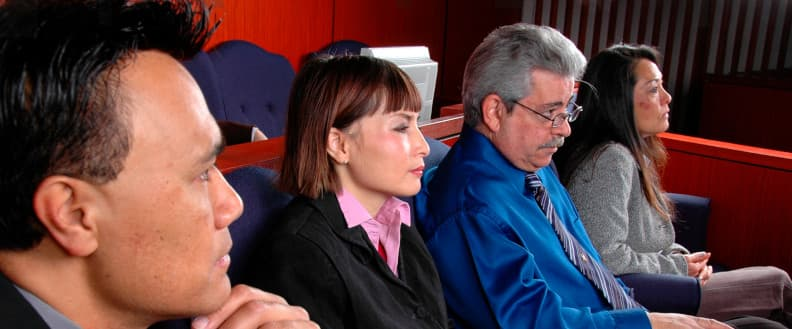# Explaining probability to a juryIn a jury of 12 people, it’s not likely that many will understand probability. In fact, it’s fairly likely that no one on a jury will have much grasp of probability.

People who have not studied probability nevertheless have some intuitive sense of probability, but they need help relating their intuitive sense to numbers and calculations. They need someone to relate numbers in unfamiliar settings to numbers in more familiar settings. They also need a guide to avoid misunderstandings.

Probability is subtle, and even people who are trained in math can fall into common errors. Two of the most common errors are (1) wrongly assuming independence and (2) getting conditional probabilities backward.

## Wrongful assumption of independence

When two events are independent, the probability of both happening is simply the product of the probability of each happening separately. For example, if you flip a fair coin, there is a 1/2 probability of the coin coming up heads. And if you roll a standard die, there’s a 1/6 probability that you’ll roll a six. Assuming there’s no way for the coin flip and the die roll to influence each other, the probability of the coin coming up heads and the die coming up six is simply 1/2 × 1/6 = 1/12.

Independence is attractive because it is easy to compute, but it is not always appropriate. For example, if there’s a 30% chance of rain today and a 40% chance of rain tomorrow, you can’t assume there’s a 12% chance that it will rain both days. The weather on one day tells you something of what to expect the next day. Weather forecasts for consecutive days are not independent.

It may be that a small, isolated storm is coming into your area. Knowing that it arrived today means there’s little chance of rain tomorrow. Or maybe there’s a huge storm system slowly approaching your area. Knowing that it arrived today means it’s likely to stay for the next day or two. In the first instance, assuming independence would over-estimate the chance of rain on both days. In the second instance, it would under-estimate the chances.

Sally Clark’s life was ruined by a wrong-headed assumption of independent probability. Her first child died of SIDS (called “cot death” in her native UK) in 1996. When her second son also died of SIDS the following year, she was accused of murdering both her children. The prosecution’s argument was that the chances of one child dying from SIDS were 1 in 8543, so the probability of two such deaths in the same family were one in 8543 × 8543 or about 73,000,000, too small to be a coincidence. This argument was persuasive and in 1999 she was convicted of murder and sent to prison.

The Royal Statistical Society (RSS) objected to this misuse of probability in 2001. The two children were brothers, sharing common genetics and a common environment. Their circumstances were not independent. Wrongfully assuming independence lead to a probability estimate that was likely much too small. The RSS also objected that the probability, even if it were correct, was being used inappropriately. In 2003 Sally Clark’s conviction was overturned. Although she was released from prison, she never recovered from her ordeal and died of alcohol poisoning in 2007.

## Backward probability calculations

When one event influences the probability of another, things become more complicated. Unfortunately, when we start discussing the probability of one event given another, there’s a tendency for people to get their calculations backward.

There’s a tendency to look at the probability of lab results assuming that someone has a disease. But that’s the opposite of what we usually want to know, the probability of having a disease assuming certain lab results.

In paternity cases one could think of the probability of certain DNA markers given that a man is the father of a child. But the issue at hand in such a case is the opposite, the probability that the man is the father given the DNA markers.

It’s easy to confuse the probability of evidence given that someone is guilty, with the probability that someone is guilty given the evidence!

Reversing probabilities can make a huge difference. For example, there’s a fair chance that a US president, selected at random, is from Ohio. But the chances that a randomly chosen person from Ohio has been president of the US are very small. People are not so likely to get these probabilities backward because both are easy to think about. But in less familiar settings, such as the probability of developing cancer after exposure to some carcinogen, it’s harder to keep probabilities straight.

## Help with probability

I have many years of experience helping people think about probability and statistics. If you’d like help thinking through probability applications and explaining the results to a jury, please call or email now to discuss your case.